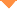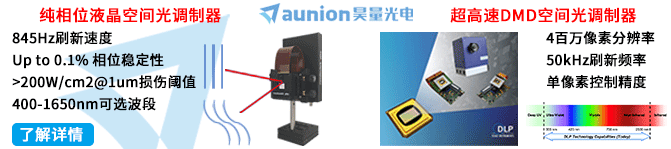首页 > 论文 > 激光与光电子学进展 > 57卷 > 13期(pp：131205--1)

Two-Frame Phase Shift Extraction Algorithm Based on Gradient Descent Algorithm

• 摘要• 论文信息• 参考文献• 被引情况• PDF全文Abstract

Two-frame phase shift extraction algorithm is characterized by small number of fringe patterns and fast processing speed. However, there are many challenges related to the design of this algorithm because of the pathological problems associated with the two-frame phase shift technology. In this study, a phase shift extraction algorithm is proposed for a two-frame random time-domain phase-shifted fringe pattern. First, the background term of the fringe pattern was eliminated using high-pass filtering. Subsequently, the minimum square root of the amplitude was searched using the gradient descent algorithm in combination with the square root distribution law of amplitude. Then, the introduced phase shift was extracted. Finally, the two-step phase shift algorithm was used to obtain the measured phase. The simulation and experimental results show that compared with other classical algorithms, the proposed algorithm exhibits various features, including small recovery phase error and noise insensitivity. Furthermore, the proposed algorithm is simple, flexible, and easy to apply.【4】Servin M, Estrada J C, Quiroga J A. The general theory of phase shifting algorithms [J]. Optics Express. 2009, 17(24): 21867-21881.

【5】Santoyo F M, Kerr D, Tyrer J R. Interferometric fringe analysis using a single phase step technique [J]. Applied Optics. 1988, 27(21): 4362-4364.

【6】Almaza''''n-Cue''''llar S. Two-step phase-shifting algorithm [J]. Optical Engineering. 2003, 42(12): 3524-3531.

【7】Zhu Y J, Liu L R, Luan Z, et al. Discussions about FFT-based two-step phase-shifting algorithm [J]. Optik. 2008, 119(9): 424-428.

【8】Xu X F, Cai L Z, Wang Y R, et al. Blind phase shift extraction and wavefront retrieval by two-frame phase-shifting interferometry with an unknown phase shift [J]. Optics Communications. 2007, 273(1): 54-59.

【9】Trusiak M, Patorski K. Two-shot fringe pattern phase-amplitude demodulation using Gram-Schmidt orthonormalization with Hilbert-Huang pre-filtering [J]. Optics Express. 2015, 23(4): 4672-4690.

【10】Zhang Y, Tian X B, Liang R G. Random two-step phase shifting interferometry based on Lissajous ellipse fitting and least squares technologies [J]. Optics Express. 2018, 26(12): 15059-15072.

【11】Zhang H Y, Zhao H, Zhao Z X, et al. Two-frame fringe pattern phase demodulation using Gram-Schmidt orthonormalization with least squares method [J]. Optics Express. 2019, 27(8): 10495-10508.

【12】Du H B, Zhang S F, Zhao Z X, et al. Development of two-frame shadow Moiré profilometry by iterative self-tuning technique [J]. Optical Engineering. 2018, 57(11): 114101.

【13】Vargas J, Quiroga J A, Belenguer T, et al. Two-step self-tuning phase-shifting interferometry [J]. Optics Express. 2011, 19(2): 638-648.

Zhang Shaofeng,Du Hubing,Guo Ruiqing,He Zhouxuan. Two-Frame Phase Shift Extraction Algorithm Based on Gradient Descent Algorithm[J]. Laser & Optoelectronics Progress, 2020, 57(13): 131205